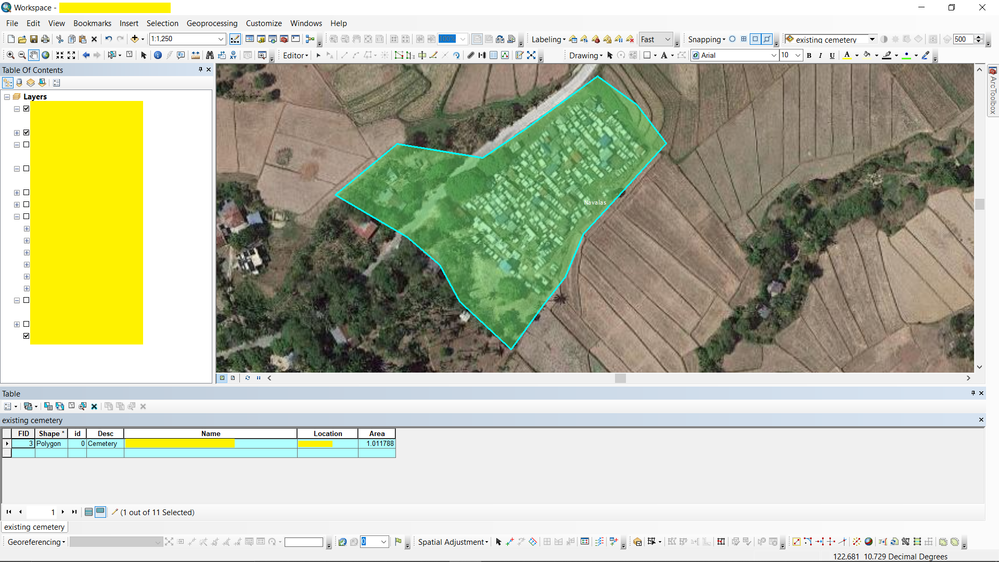# Automatically Resizing Polygons

802
6
09-08-2021 10:34 PMby
New Contributor II

Hello everyone,

I'm currently using ArcGIS 10.5. I was wondering if there is a tool that automatically adjusts a polygon to a specified size? Say I start with a triangle of 3 hectares, and I want to increase its area in all directions for it to become 5 hectares automatically, is there a tool that does this? Or a combination of tools perhaps?

Thank you

Tags (3)
1 Solution

Accepted SolutionsbyEsri Contributor

I did come across this bit of code, which may help:

```import arcpy
import math

def scale_geom(geom, scale, reference=None):
"""Returns geom scaled to scale %"""
if geom is None: return None
if reference is None:
# we'll use the centroid if no reference point is given
reference = geom.centroid

refgeom = arcpy.PointGeometry(reference)
newparts = []
for pind in range(geom.partCount):
part = geom.getPart(pind)
newpart = []
for ptind in range(part.count):
apnt = part.getObject(ptind)
if apnt is None:
# polygon boundaries and holes are all returned in the same part.
# A null point separates each ring, so just pass it on to
# preserve the holes.
newpart.append(apnt)
continue
bdist = refgeom.distanceTo(apnt)

bpnt = arcpy.Point(reference.X + bdist, reference.Y)
cdist = arcpy.PointGeometry(apnt).distanceTo(bpnt)

# Law of Cosines, angle of C given lengths of a, b and c
angle = math.acos((adist**2 + bdist**2 - cdist**2) / (2 * adist * bdist))

scaledist = bdist * scale

# If the point is below the reference point then our angle
# is actually negative
if apnt.Y < reference.Y: angle = angle * -1

# Create a new point that is scaledist from the origin
# along the x axis. Rotate that point the same amount
# as the original then translate it to the reference point
scalex = scaledist * math.cos(angle) + reference.X
scaley = scaledist * math.sin(angle) + reference.Y

newpart.append(arcpy.Point(scalex, scaley))
newparts.append(newpart)

return arcpy.Geometry(geom.type, arcpy.Array(newparts), geom.spatialReference)```

You can call it with a geometry object, a scale factor (1 = same size, 0.5 = half size, 5 = 5 times as large, etc.), and an optional reference point:

`scale_geom(some_geom, 1.5)`

Referenced from here:

https://gis.stackexchange.com/questions/169694/is-there-arcpy-tool-for-polygon-resizing-like-scale-t...

Regards,
Mark
6 RepliesbyEsri Contributorby
New Contributor II

Dear Mark,

Thanks for the response!

Although my problem with this tool is that I don't seem to end up with the exact measurement I want. You will see in the first image that the polygon is 0.890589 hectares. I wanted to upscale this to 0.9494 hectares by using 1.06571298 as the Scale Factor. You will see in the next image that I didn't arrive at this and instead got 1.011788.  I'm not sure what's happening here but this tool can't seem to help me with what I want to happen. Any other solutions you can suggest?Before Scale ToolAfter Scale ToolbyEsri Contributor

I am unsure why the tool behaves in that way. It might be worth raising a support ticket to see if there something that can be done.

I cant' think of any other OOTB tools that can help you with your problem. You could probably code something up in Python to do the job though.

Regards,
Markby
New Contributor II

Hello again Mark,

Maybe I can bring it up with them. Unfortunately, I don't know enough Python to construct a code that will execute said task. I may have to wait for a response from esri regarding the tool.byEsri Contributor

I did come across this bit of code, which may help:

```import arcpy
import math

def scale_geom(geom, scale, reference=None):
"""Returns geom scaled to scale %"""
if geom is None: return None
if reference is None:
# we'll use the centroid if no reference point is given
reference = geom.centroid

refgeom = arcpy.PointGeometry(reference)
newparts = []
for pind in range(geom.partCount):
part = geom.getPart(pind)
newpart = []
for ptind in range(part.count):
apnt = part.getObject(ptind)
if apnt is None:
# polygon boundaries and holes are all returned in the same part.
# A null point separates each ring, so just pass it on to
# preserve the holes.
newpart.append(apnt)
continue
bdist = refgeom.distanceTo(apnt)

bpnt = arcpy.Point(reference.X + bdist, reference.Y)
cdist = arcpy.PointGeometry(apnt).distanceTo(bpnt)

# Law of Cosines, angle of C given lengths of a, b and c
angle = math.acos((adist**2 + bdist**2 - cdist**2) / (2 * adist * bdist))

scaledist = bdist * scale

# If the point is below the reference point then our angle
# is actually negative
if apnt.Y < reference.Y: angle = angle * -1

# Create a new point that is scaledist from the origin
# along the x axis. Rotate that point the same amount
# as the original then translate it to the reference point
scalex = scaledist * math.cos(angle) + reference.X
scaley = scaledist * math.sin(angle) + reference.Y

newpart.append(arcpy.Point(scalex, scaley))
newparts.append(newpart)

return arcpy.Geometry(geom.type, arcpy.Array(newparts), geom.spatialReference)```

You can call it with a geometry object, a scale factor (1 = same size, 0.5 = half size, 5 = 5 times as large, etc.), and an optional reference point:

`scale_geom(some_geom, 1.5)`

Referenced from here:

https://gis.stackexchange.com/questions/169694/is-there-arcpy-tool-for-polygon-resizing-like-scale-t...

Regards,
Markby
New Contributor II

Hello Mark!

Thanks for this, although I've found another workaround (albeit manual) solution for my problem, I'll surely be studying this.

All the best! :D